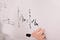# Eigenvectors and Eigenvalues: A deeper understanding

The literal meaning of “Eigen” is characteristic which comes from the German language. These vectors are aptly named as these vectors ultimately give the trend something follows. Any matrix that has an eigenvector, all its coordinates will ultimately follow a relation as close to the eigenvector as possible. To expand on this I will be using some examples.

# The Fibonacci Sequence

To explain the Fibonacci sequence, it is just the sum of the previous two terms. If we start the sequence with 0 and 1 the sequence would be

0, 1, 1, 2, 3, 5, 8, 13…

and it goes on till infinity…

# Solutions to Ordinary Differential Equations of First OrderPhoto by Jeswin Thomas on Unsplash

There are so many aspects a person has to keep in mind while solving differential equations that you’re bound to forget something at some point. This can be very frustrating as by making a small mistake, differential equations can become unsolvable. The purpose for writing this is to create a helping guide to help remember everything.

# Variable Separable ODEs

I’ll start with variable separable differential equations. Although a variable separable ODE should be in the form

dy/dx = g(x)h(y)

which you can easily solve by applying algebraic operations and converting it into the form

h(y)dy = g(x)dx

and then finally integrating both sides…